+关注继续查看

# 第2章

• 深度学习平台概述。
• 搭建图像识别开发环境。
• Numpy的使用详解。

## 2.1　深度学习框架

### 2.1.1　Theano

Theano是在BSD许可证下发布的一个开源项目，是由LISA集团（现MILA）在加拿大魁北克的蒙特利尔大学开发的，其是以一位希腊数学家的名字命名的。
Theano是一个Python库，可用于定义、优化和计算数学表达式，特别是多维数组（numpy.ndarray）。它的诞生是为了执行深度学习中的大规模神经网络算法，从本质上而言，Theano可以被理解为一个数学表达式的编译器：用符号式语言定义程序员所需的结果，并且Theano可以高效地运行于GPU或CPU中。

### 2.1.2　Tensorflow

Tensorflow在很大程度上可以看作是Theano的后继者，不仅因为它们有很大一批共同的开发者，而且它们还拥有相近的设计理念：它们都是基于计算图实现自动微分系统。Tensorflow使用数据流图进行数值计算，图中的节点代表数学运算，图中的边则代表在这些节点之间传递的多维数组（tensor）。

• 由于Tensorflow的接口一直处于快速迭代之中，并且版本之间存在不兼容的问题，因此开发和调试过程中可能会出现一些问题（许多开源代码无法在新版的Tensorflow上运行）。
• 想要学习Tensorflow底层运行机制的读者需要做好准备，Tensorflow在GitHub代码仓库的总代码量超过100万行，系统设计比较复杂，因此这将是一个漫长的过程。
• 代码层面，对于同一个功能，Tensorflow提供了多种实现，这些实现良莠不齐，使用中还存在细微的区别，请读者注意，避免入坑。另外，Tensorflow还创造了图、会话、命名空间、PlaceHolder等诸多抽象概念，对普通用户来说可能会难以理解。

### 2.1.3　MXNet

MXNet是亚马逊（Amazon）的李沐带队开发的深度学习框架。它拥有类似于Theano和Tensorflow的数据流图，为多GPU架构提供了良好的配置，有着类似于Lasagne和Blocks的更高级别的模型构建块，并且可以在你想象的任何硬件上运行（包括手机）。对Python的支持只是其功能的冰山一角，MXNet同样提供了对R、Julia、C++、Scala、Matlab、Golang和Java的接口。
MXNet以其超强的分布式支持，明显的内存、显存优化为人所称道。同样的模型，MXNet往往占用更小的内存和显存，并且在分布式环境下，MXNet展现出了明显优于其他框架的扩展性能。
MXNet的缺点是推广不给力及接口文档不够完善。MXNet长期处于快速迭代的过程中，其文档却长时间未更新，这就导致新手用户难以掌握MXNet，老用户则需要常常查阅源码才能真正理解MXNet接口的用法。

### 2.1.4　Keras

Keras是一个高层神经网络API，由纯Python语言编写而成，并使用Tensorflow、Theano及CNTK作为后端。Keras为支持快速实验而生，能够将想法迅速转换为结果。Keras应该是深度学习框架之中最容易上手的一个，它提供了一致而简洁的API，能够极大地减少一般应用下用户的工作量，避免用户重复造轮子，而且Keras支持无缝CPU和GPU的相互转换。

### 2.1.5　PyTorch

PyTorch是一个Python优先的深度学习框架，能够在强大的GPU加速的基础上实现张量和动态神经网络。
PyTorch是一个Python软件包，其提供了两种高层面的功能，具体如下。
1）使用强大的GPU加速的Tensor计算（类似于Numpy）。

### 2.1.6　Caffe

Caffe是早期深度学习研究者使用的框架，由于很多研究人员在上面进行开发和优化，因此其现今也是流行的框架之一。Caffe也存在不支持多机、跨平台、可扩展性差等问题。初学者使用Caffe时还需要注意下面这些问题。
1）Caffe的安装过程需要大量的依赖库，因此会涉及很多安装版本问题，初学者不易上手。
2）当用户想要实现一个新的层时，需要用C++实现它的前向传播和反向传播代码，而如果想要新层运行在GPU之上，则需要同时使用CUDA实现这一层的前向传播和反向传播。

    Caffe2：https://caffe2.ai/ 

## 2.2　搭建图像识别开发环境

### 2.2.1　Anaconda

• 包管理：不同的包在安装和使用的过程中都会遇到版本匹配和兼容性等问题，在实际工程中经常会使用大量的第三方安装包，若人工手动进行匹配是非常耗时耗力的事情，因此包管理是非常重要的内容。
• 环境管理：用户可以使用conda来创建虚拟环境，其可以很方便地解决多版本Python并存、切换等问题。Mac上安装完Anaconda之后，在应用程序界面里就能看到Anaconda Navigator的图标，点击运行之后就能看到如图2-2所示的界面，然后选Notebook，点击“Launch”按钮，浏览器中会出现如图2-3所示的画面。Windows可以从“开始”菜单中找到Anaconda，然后点击Jupyter Notebook运行。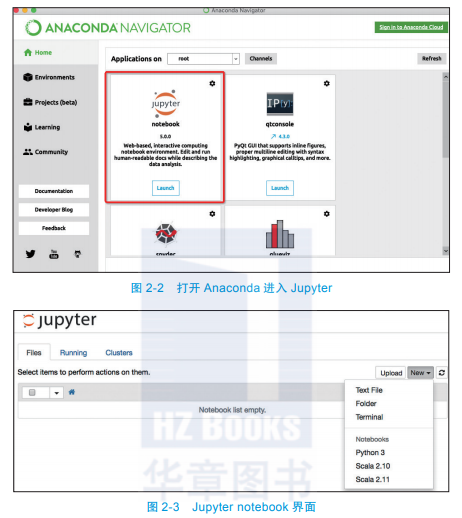JupyterNotebook提供的功能之一就是可以使我们多次编辑Cell（代码单元格），在实际开发当中，为了得到最好的效果，我们往往会对测试数据（文本）使用不同的技术进行解析与探索，因此Cell的迭代分析数据功能变得特别有用。

### 2.2.2　conda

1.包的安装和管理
conda对包的管理都是通过命令行来实现的（Windows用户可以参考面向Windows的命令提示符教程），若想要安装包，那么在终端中输入conda install package_name即可。例如，要安装Numpy，输入如下代码：

conda install numpy

conda还会自动为你安装依赖项。例如，scipy依赖于Numpy，如果你只安装scipy（conda install scipy），则conda还会安装Numpy（如果尚未安装的话）。
conda的大多数命令都是很直观的。要卸载包，请使用conda remove package_name；要更新包，请使用conda update package_name。如果想更新环境中的所有包（这样做常常很有用），请使用conda update --all。最后，要想列出已安装的包，请使用前面提过的conda list。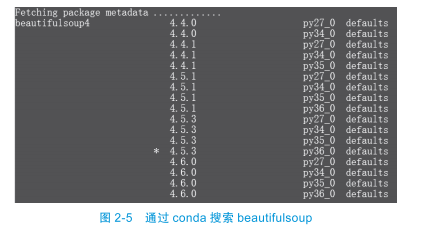2.环境管理

#创建第2~7章代码运行的环境：
conda create -n basic_env  python=3.7    #创建一个名为basic_env的环境
source activate basic_env        #激活这个环境—Linux和macOS代码
activate basic_env            #激活这个环境—Windows代码
#创建第8~12章代码运行的环境：
conda create -n imgrecognition_env  python=3.7
#创建一个名为imgrecognition _env的环境
source activate imgrecognition _env    #激活这个环境—Linux和macOS代码
activate imgrecognition_env        #激活这个环境—Windows代码

### 2.2.3　Pytorch的下载与安装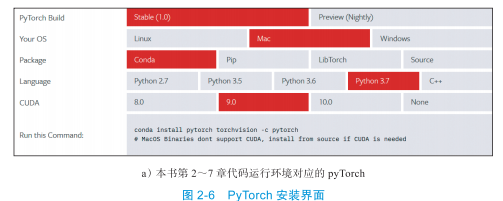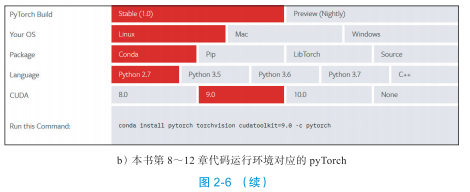## 2.3　Numpy使用详解

Numpy（Numerical Python的简称）是高性能科学计算和数据分析的基础包，其提供了矩阵运算的功能。Numpy提供的主要功能具体如下。

• ndarray—一个具有向量算术运算和复杂广播能力的多维数组对象。
• 用于对数组数据进行快速运算的标准数学函数。
• 用于读写磁盘数据的工具以及用于操作内存映射文件的工具。
• 非常有用的线性代数，傅里叶变换和随机数操作。
• 用于集成C /C++和Fortran代码的工具。

### 2.3.1　创建数组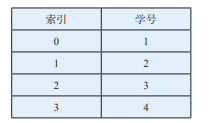1）在Anaconda的Notebook里输入import numpy as np之后，通过键盘按住Shift+Enter执行，如果没有报错，则说明Numpy已被正常引入，如图2-7所示。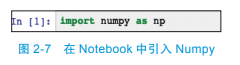2）Numpy中的array()可以直接导入向量，代码如下：

vector = np.array([1,2,3,4])

3）numpy.array()方法也可以导入矩阵，代码如下：

matrix = np.array([[1,'Tim'],[2,'Joey'],[3,'Johnny'],[4,'Frank']])

### 2.3.2　创建Numpy数组

Numpy数组还封装了其他方法来创建矩阵。首先，我们介绍第一个方法np.zeros（从命名规则来看，这个方法就是用来创建数值都为0的向量），比如，我们输入：

a = np.zeros(10)

array([ 0.,  0.,  0.,  0.,  0.,  0.,  0.,  0.,  0.,  0.])

np.zeros(10,dtype=int)

np.zeros(shape=(3,4)) #代表创建的是三行四列的矩阵并且其数据类型为float64

array([[ 0.,  0.,  0.,  0.],
[ 0.,  0.,  0.,  0.],
[ 0.,  0.,  0.,  0.]])

np.ones((3,4))

array([[ 1.,  1.,  1.,  1.],
[ 1.,  1.,  1.,  1.],
[ 1.,  1.,  1.,  1.]])

np.full((3,5),121) #这个方法的意思是我们创建了一个三行五列的矩阵，默认值为121

array([[121, 121, 121, 121, 121],
[121, 121, 121, 121, 121],
[121, 121, 121, 121, 121]])

np.arange(0,20,2) #arange接收三个参数，与Python中的range方法相似，arange也是前闭后开的方法，第一个参数为向量的第一个值0，第二个参数为最后一个值20，因为是后开所以取的是18，第三个参数为步长，默认为1，本例中设置为2，所以最后一个值是18。

array([ 0,  2,  4,  6,  8, 10, 12, 14, 16, 18])

np.linspace(0,10,5)

array([  0. ,   2.5,   5. ,   7.5,  10. ])

1）生成一个长度为10的向量，里面每一个数值都是介于0～10之间的整数，代码如下：

import numpy as np
np.random.randint(0,10,10)

2）如果不确定每个参数代表的意思，则加上参数名size，代码如下：

np.random.randint(0,5,size=5)  #注意是前闭后开，永远取不到5

3）我们也可以生成一个三行五列的整数矩阵，代码如下

np.random.randint(4,9,size=(3,5))

4）seed的作用：如果不希望每次生成的随机数都不固定，那么我们可以使用np.random.seed(1)，随机种子使用数字1记录，这以后只要是用随机种子1生成的随机数就都是固定的。
5）我们也可以生成介于0～1之间的浮点数的向量或者矩阵，代码如下：

np.random.random(10)        #生成0~1之间的浮点数，向量的长度为10
np.random.random((2,4))        #生成0~1之间的浮点数，二行四列的矩阵

6）np.random.normal()表示的是一个正态分布，normal在这里是正态的意思。numpy.random.normal(loc=0,scale=1,size=shape)的意义如下。

• 参数loc(float)：正态分布的均值，对应这个分布的中心。loc=0说明这是一个以Y轴为对称轴的正态分布。
• 参数scale(float)：正态分布的标准差，对应分布的宽度，scale越大，正态分布的曲线越矮胖，scale越小，曲线越高瘦。
• 参数size(int或者整数元组)：输出的值赋在shape里，默认为None。

### 2.3.3　获取Numpy属性

a = np.arange(15).reshape(3,5)        #代表3行5列

array([[ 0,  1,  2,  3,  4],
[ 5,  6,  7,  8,  9],
[10, 11, 12, 13, 14]])


print(a.shape)

(3, 5)

importnumpy as np
x = np.arange(15)
print(x.ndim)        #输出x向量的维度，这时能看到的维度是1维
X = x.reshape(3,5)    #将x向量转为三行五列的二维矩阵
Print(X.ndim)        #输出X矩阵的维度，这时能看到的维度是2维

reshape方法的特别用法

x.reshape(15,-1)    #我关心的是我只要15行，列由计算机自己来算
x.reshape(-1,15)    #我关心的是我只要15列，行由计算机自己来算

### 2.3.4　Numpy数组索引

Numpy支持类似list的定位操作，示例代码如下：

import numpy as np
matrix = np.array([[1,2,3],[20,30,40]])
print(matrix[0,1])

### 2.3.5　切片

Numpy支持类似list的切片操作，示例代码如下：

import numpy as np
matrix = np.array([
[5, 10, 15],
[20, 25, 30],
[35, 40, 45]
])
print(matrix[:,1])
print(matrix[:,0:2])
print(matrix[1:3,:])
print(matrix[1:3,0:2])

print(matrix[:,0:2])代表的是选取所有的行，而且列的索引是0和1的数据。
print(matrix[1:3,:])代表的是选取所有的列，而且行的索引值是1和2的数据。
print(matrix[1:3,0:2])代表的是选取行的索引是1和2，而且列的索引是0和1的所有数据。

### 2.3.6　Numpy中的矩阵运算

import numpy as np
myones = np.ones([3,3])
myeye = np.eye(3)        #生成一个对角线的值为1，其余值都为0的三行三列矩阵
print(myeye)
print(myones-myeye)

[[ 1.  0.  0.]
[ 0.  1.  0.]
[ 0.  0.  1.]]
[[ 0.  1.  1.]
[ 1.  0.  1.]
[ 1.  1.  0.]]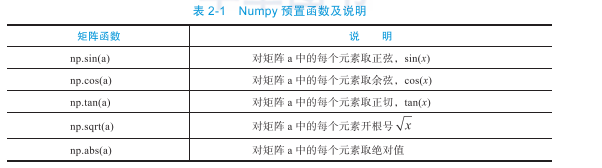（1）矩阵之间的点乘

import numpy as np
mymatrix = np.array([[1,2,3],[4,5,6]])
a = np.array([[1,2],[3,4],[5,6]])
print(mymatrix.shape == a.shape)
print(mymatrix.dot(a))

[[22 28]
[49 64]]

（2）矩阵的转置

import numpy as np
a = np.array([[1,2,3],[4,5,6]])
print(a.T)

[[1 4]
[2 5]
[3 6]]

（3）矩阵的逆

import numpy as np
import numpy.linalg as lg
A = np.array([[0,1],[2,3]])
invA = lg.inv(A)
print(invA)
print(A.dot(invA))

[[-1.5  0.5]
[ 1.   0. ]]

### 2.3.7　数据类型转换

Numpy ndarray数据类型可以通过参数dtype进行设定，而且还可以使用参数astype来转换类型，在处理文件时该参数会很实用。注意，astype调用会返回一个新的数组，也就是原始数据的备份。

vector = numpy.array(["1", "2", "3"])
vector = vector.astype(float)


### 2.3.8　Numpy的统计计算方法

NumPy内置了很多计算方法，其中最重要的统计方法及说明具体如下。

• sum()：计算矩阵元素的和；矩阵的计算结果为一个一维数组，需要指定行或者列。
• mean()：计算矩阵元素的平均值；矩阵的计算结果为一个一维数组，需要指定行或者列。
• max()：计算矩阵元素的最大值；矩阵的计算结果为一个一维数组，需要指定行或者列。
• mean()：计算矩阵元素的平均值。
• median()：计算矩阵元素的中位数。

vector = numpy.array([5, 10, 15, 20])
vector.sum()

matrix=
array([[ 5, 10, 15],
[20, 10, 30],
[35, 40, 45]])
matrix.sum(axis=1)
array([ 30,  60, 120])
matrix.sum(axis=0)
array([60, 60, 90])

### 2.3.9　Numpy中的arg运算

argmax函数就是用来求一个array中最大值的下标。简单来说，就是最大的数所对应的索引（位置）是多少。示例代码如下：

index2 = np.argmax([1,2,6,3,2])        #返回的是2

argmin函数可用于求一个array中最小值的下标，用法与argmax类似。示例代码如下：

index2 = np.argmin([1,2,6,3,2]) #返回的是0

import numpy as np
x = np.arange(15)
print(x)    # array([ 0,  1,  2,  3,  4,  5,  6,  7,  8,  9, 10, 11, 12, 13, 14])
np.random.shuffle(x)    #随机打乱
print(x)    # array([ 8, 13, 12,  3,  9,  2, 10,  0, 11,  5, 14,  7,  1,  4,  6])
sx = np.argsort(x)    #从小到大排序，返回索引值
print(sx)    # [ 7 12  5  3 13  9 14 11  0  4  6  8  2  1 10]

### 2.3.10　FancyIndexing

import numpy as np
x = np.arange(15)
ind = [3,5,8]
print(x[ind]) #使用fancyindexing就可以解决这个问题

import numpy as np
x = np.arange(15)
np.random.shuffle(x)
ind = np.array([[0,2],[1,3]])  #第一行需要取x向量中索引为0的元素，以及索引为2的元素，
第二行需要取x向量中索引为1的元素以及索引为3的元素
print(x)
print(x[ind])

[ 3  2  7 12  9 13 11 14 10  5  4  1  6  8  0]
[[ 3  7]
[ 2 12]]

import numpy as np
x = np.arange(16)
X = x.reshape(4,-1)
row = np.array([0,1,2])
col = np.array([1,2,3])
print(X[row,col])        #相当于取三个点，分别是(0,1),(1,2),(2,3)
print(X[1:3,col])        #相当于取第2、3行，以及需要的列

### 2.3.11　Numpy数组比较

Numpy有一个强大的功能是数组或矩阵的比较，数据比较之后会产生boolean值。示例代码如下：

import numpy as np
matrix = np.array([
[5, 10, 15],
[20, 25, 30],
[35, 40, 45]
])
m = (matrix == 25)
print(m)

[[False False False]
[False  True False]
[False False False]]

import numpy as np
matrix = np.array([
[5, 10, 15],
[20, 25, 30],
[35, 40, 45]
])
second_column_25 =  (matrix[:,1] == 25)
print(second_column_25)
print(matrix[second_column_25, :])

## 2.4　本章小结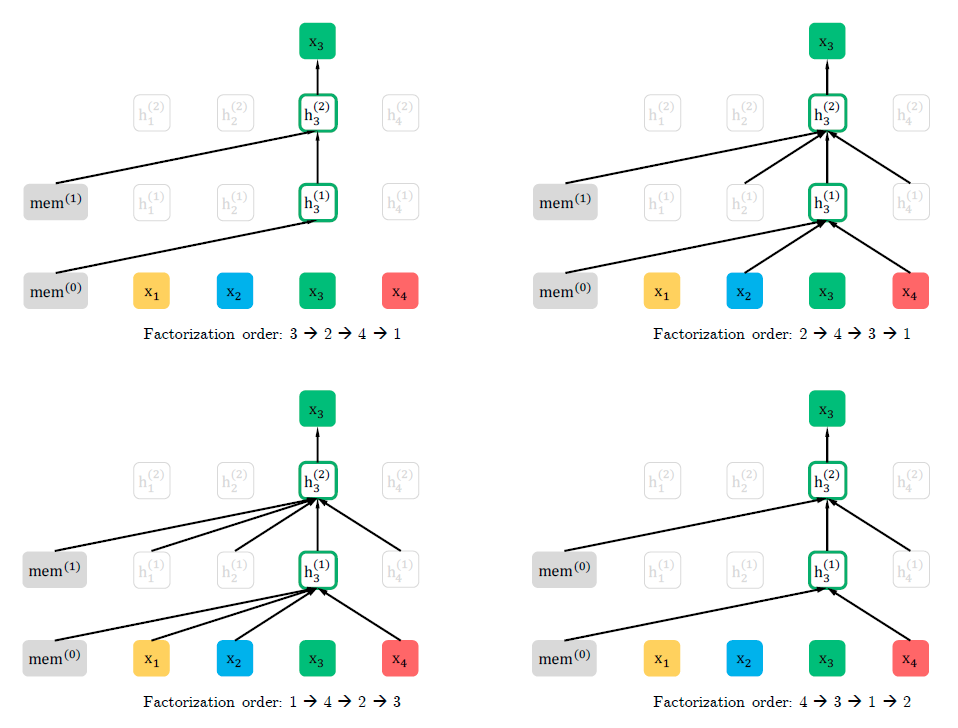37 0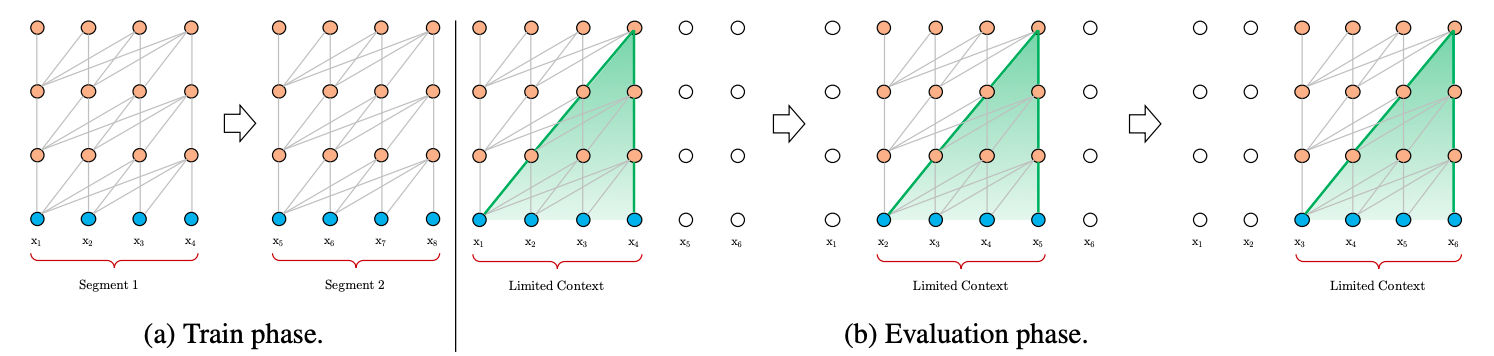69 0122 0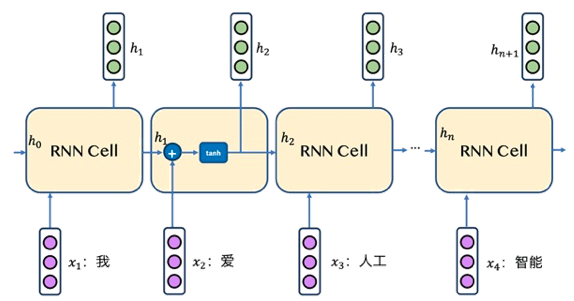41 0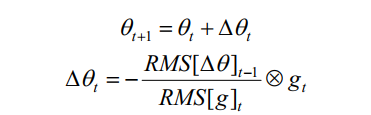【深度学习】1、优化方法原理与实践对比——非常详细与全面（二）
【深度学习】1、优化方法原理与实践对比——非常详细与全面（二）
13 0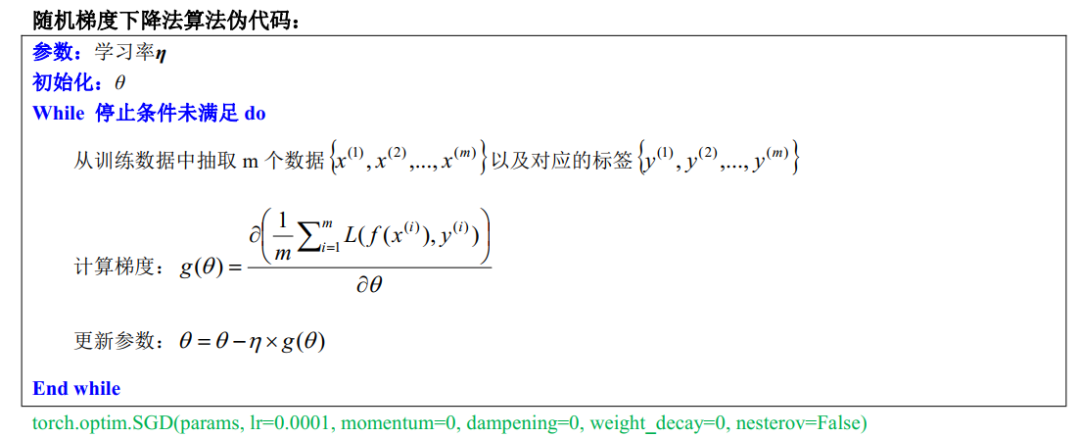【深度学习】1、优化方法原理与实践对比——非常详细与全面（一）
【深度学习】1、优化方法原理与实践对比——非常详细与全面（一）
18 0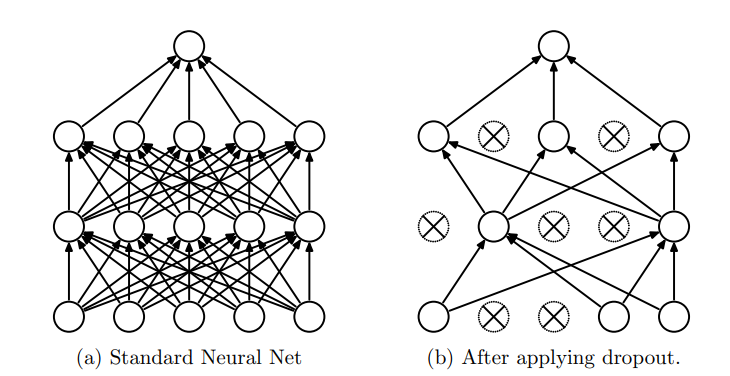【深度学习】Dropout原理以及代码实现
【深度学习】Dropout原理以及代码实现
32 0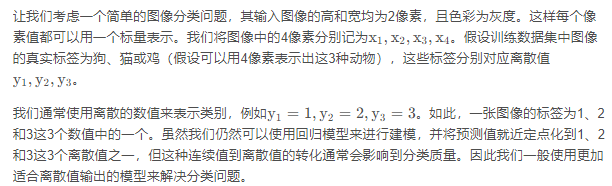【从零开始学习深度学习】5.用于分类问题的softmax回归模型原理简介
【从零开始学习深度学习】5.用于分类问题的softmax回归模型原理简介
56 0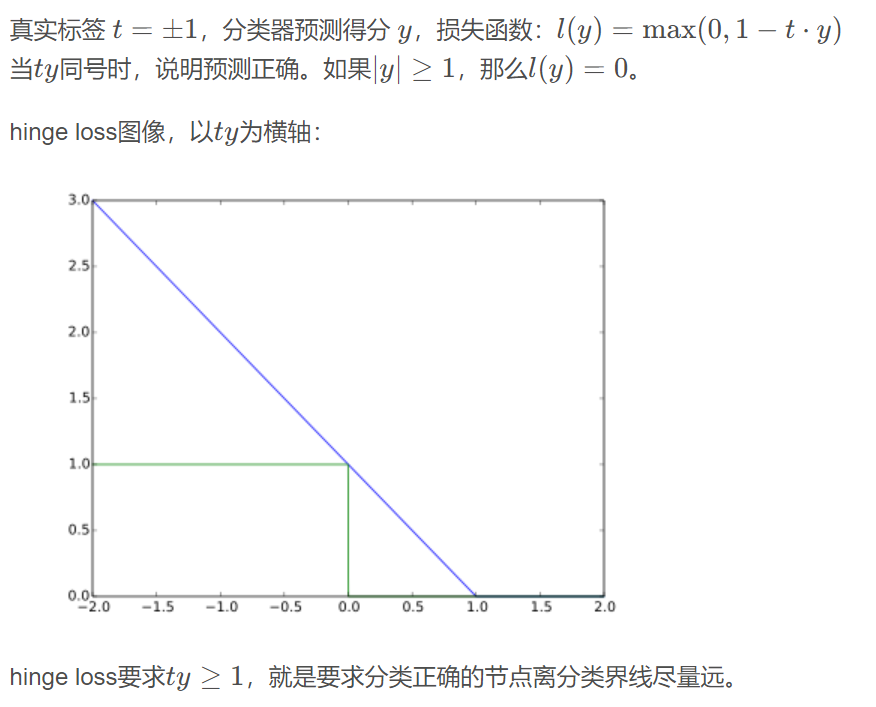183 0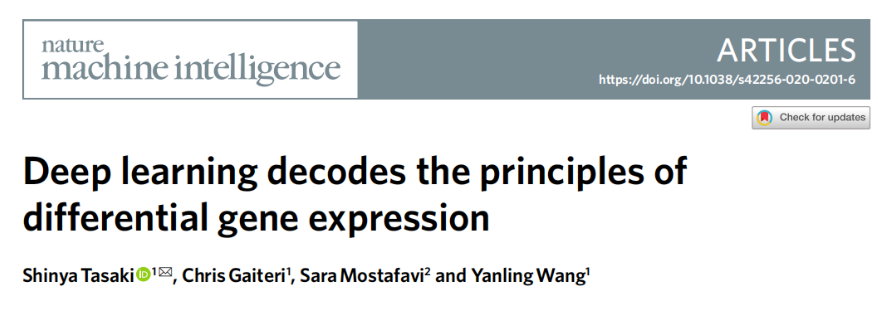Nat.Mach.Intell.| DEcode:深度学习解读差异基因表达原理
Nat.Mach.Intell.| DEcode:深度学习解读差异基因表达原理
77 0

+ 订阅

【算法实战】1. 机器学习基础2103